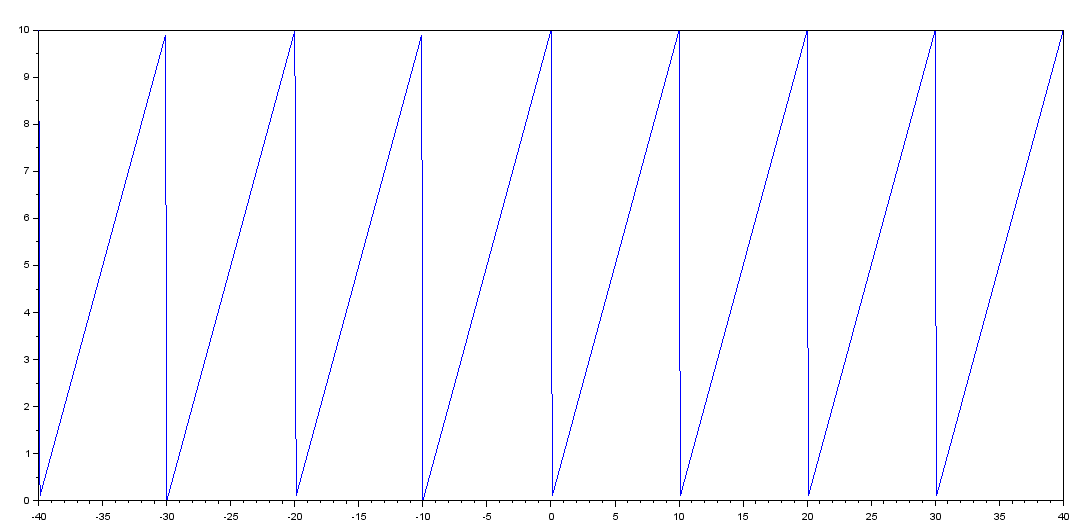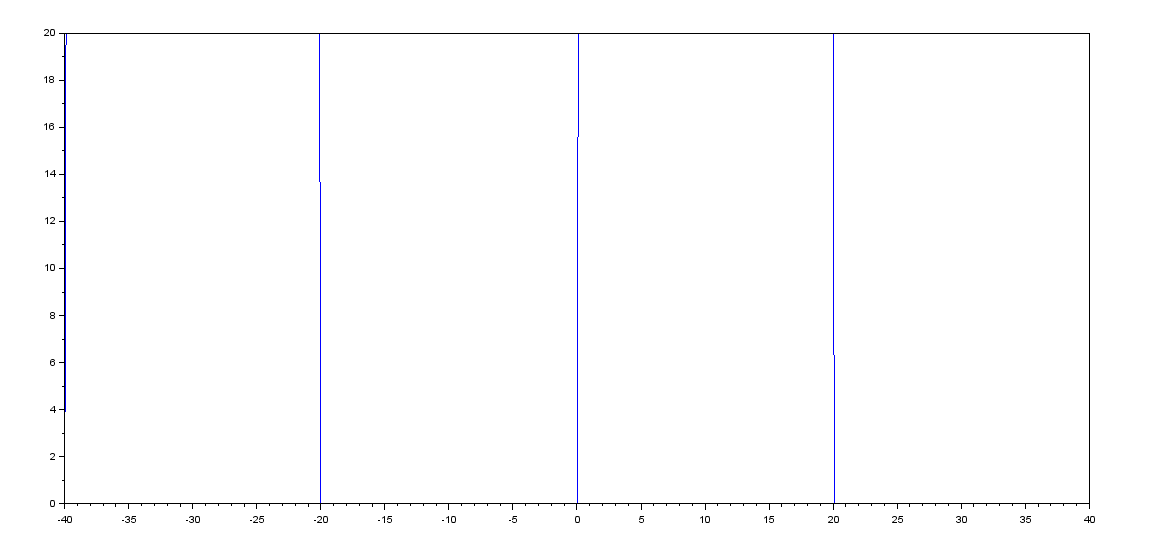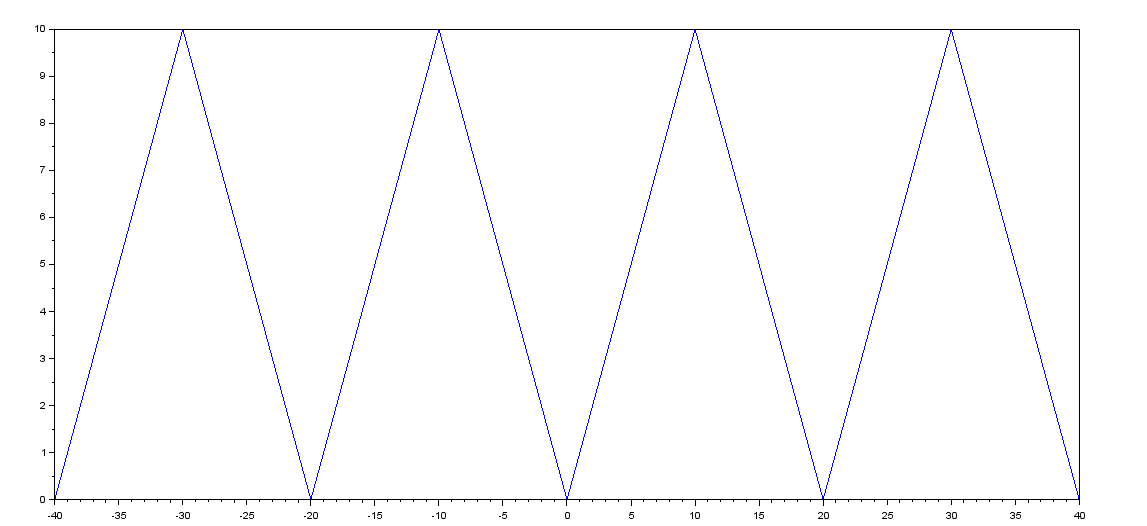# Defining a Periodic Function in SCILAB

While working on Fourier Series or some other Mathematical Problem, you might sometime have to work with Periodic Functions.

Periodic Functions are those that give the same value after a particular period.
So we will use this definition to define a periodic function in SCILAB.

Let’s say that there is a function f(x) which is periodic with a period of 2*T and is already defined in the interval [-T,T].

Then the function should have the same value at: f(x), f(x+2*T), f(x+4*T), ….

i.e. f(x)=f(x+2*T)=f(x+4*T)=……. since period=2*T.

But I said that the function is defined only in the interval [-T,T]. So how is the computer supposed to calculate it’s value at x>T?
That’s easy. Since the value of the function at f(x+2*T) is simply f(x), therefore we can generalize that whenever x>T: then,
f(x)=f(x-2*T).  Note: We have to keep taking x back by 2*T i.e (x-2*T) until it lies  within [-T,T] where the function is well-defined.

Similarly what about the value of function at x less than (-T) cause the function is not defined for values less than (-T)?
Again, this time we use:f(x)=f(x+2*T). Note: We keep translating x forward by 2*T  i.e (x+2*T) until it lies  within [-T,T] where the function is well-defined.

Using the above two arguments we can create a function which will make any given function defined within [-T,T] and with a period 2*T a periodic function.

Here is the code for that:

```//Periodic Function
function a=periodicf(T,f,x)
if (x>=-T)&(x<=T) then
a=f(x);
elseif x>T then
x_new=x-2*T;
a=periodicf(T,f,x_new);
elseif x<(-T) then
x_new=x+2*T;
a=periodicf(T,f,x_new);
end
endfunction
```

Demo:

```deff('a=f(x)','a=x');
-->periodicf(2,f,0)
ans  =
0.

-->periodicf(2,f,1)
ans =

1.

-->periodicf(2,f,2)
ans =

2.

-->periodicf(2,f,3)
ans =

- 1.

-->periodicf(2,f,4)
ans =

0.```

In the above example I have created a function f(x)=x.
Then I called the function 'periodicf' with T=2(meaning period is 4) and passed the function 'f' as the second argument and then the third argument is the value of x at which I want the value of 'f'.

The above code assumes the function to be defined within [-T,T] therefore the function's starting point is (-T).
However, if you want to create a function that is defined in a different manner then you will have to define 'f' correspondingly.

Following are some examples of different kinds of periodic functions along-with their plots:

Saw-tooth Wave(1):
Code:

```//Saw-Tooth Wave(all Positive)
funcprot(0);
function a=sawtooth(T,x)
deff('a=sawtooth_temp(x)','if x>0 then; a=x; else; a=x+T; end;');
a=periodicf(T,sawtooth_temp,x);
endfunction
//Plotting a saw-tooth wave
x=[-40:0.1:40];
for i=1:801
y(i)=sawtooth(5,x(i));
end
plot(x,y);

```

Output:Saw-Tooth(2) Wave:

Code:

```//Sawtooth wave(2)
funcprot(0);
function a=sawtooth2(T,x)
deff('a=sawtooth_temp(x)','a=x');
a=periodicf(T,sawtooth_temp,x);
endfunction
//Plotting a saw-tooth wave
x=[-40:0.1:40];
for i=1:801
y(i)=sawtooth2(10,x(i));
end
plot(x,y);

```

Output:Square Wave(or Rectangle Wave) :

Code:

```//Square-Wave or Rectangle Wave
funcprot(0);
function a=sqrwave(T,A,x)
deff('a=sqwavetmp(x)','if x>0 then; a=A; else; a=0; end')
a=periodicf(T,sqwavetmp,x);
endfunction
//Plotting a square wave
x=[-40:0.1:40];
for i=1:801
y(i)=sqrwave(20,20,x(i));
end
plot(x,y);

```

Output:Triangular Wave:

Code:

```//Triangle Wave:
funcprot(0);
function a=trianglewave(T,x)
deff('a=trnglewavetmp(x)','if x>0 then; a=x; else; a=-x; end')
a=periodicf(T,trnglewavetmp,x);
endfunction
//Plotting a Triangle wave
x=[-40:0.1:40];
for i=1:801
y(i)=trianglewave(10,x(i));
end
plot(x,y);

```

Output:[wpedon id="7041" align="center"]

## 2 thoughts on “Defining a Periodic Function in SCILAB”

1. I did know the Youtube channel but ain’t Bragitoff. Thanks a lot, you spared me a new night spent to figure out how to get a saw-tooth.

2. Hello, your codes are not explained properly. Can you explain it more? I am a beginner in sci-lab.

What is the use of “periodicf” function in second example?